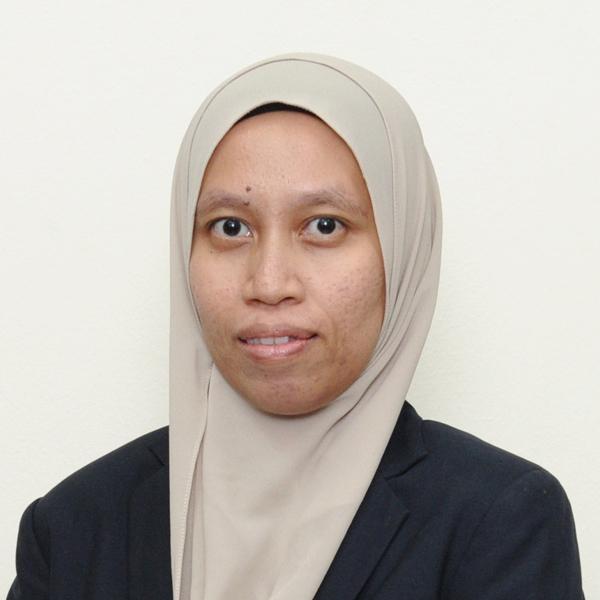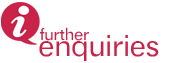﻿﻿﻿ Mathematical Physics And Engineering | INSTITUTE FOR MATHEMATICAL RESEARCH
» POSTGRADUATE » Fields of Study and Supervisors » Mathematical Physics and Engineering

# Mathematical Physics and Engineering

Mathematical Physics and Engineering is broadly the study of mathematical structures and techniques in physical sciences and engineering. The scope of study covers traditional areas of applied mathematics such as equations of fluid dynamics, continuum mechanics, and dynamical systems, as well as those from quantum sciences and relativity. The latter includes exploring novel mathematical structures that underlie quantum theory and space-time, and established topics of operator theory, equations of mathematical physics, and geometric and topological methods. Topical areas of interest are problems of cracks, integro-differential equations, foundations of quantum theory, quantum information and cosmological solutions.

List of Supervisors

 1.Assoc. Prof. Dr. Hishamuddin ZainuddinTel: +603-9769.6655Email: hisham@upm.edu.my 2.Dr. Chan Kar TimTel: +603.8946.7932Email: chankt@upm.edu.myLink:Scopus | Google Scholar | ResearchGate 3.Dr. Nurisya Mohd Shah Tel: +603.8946.6669Email: risya@upm.edu.myLink:Scopus | Google Scholar | ResearchGate

Updated:: 12/08/2020 [noorizzati]

MEDIA SHARING